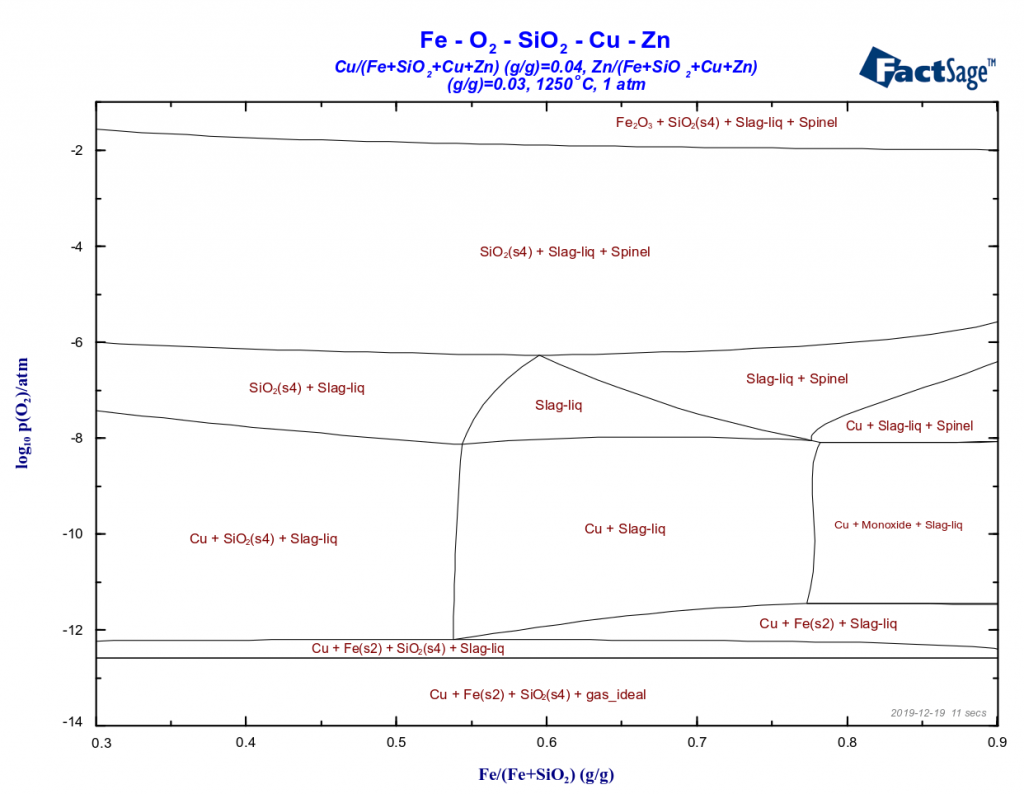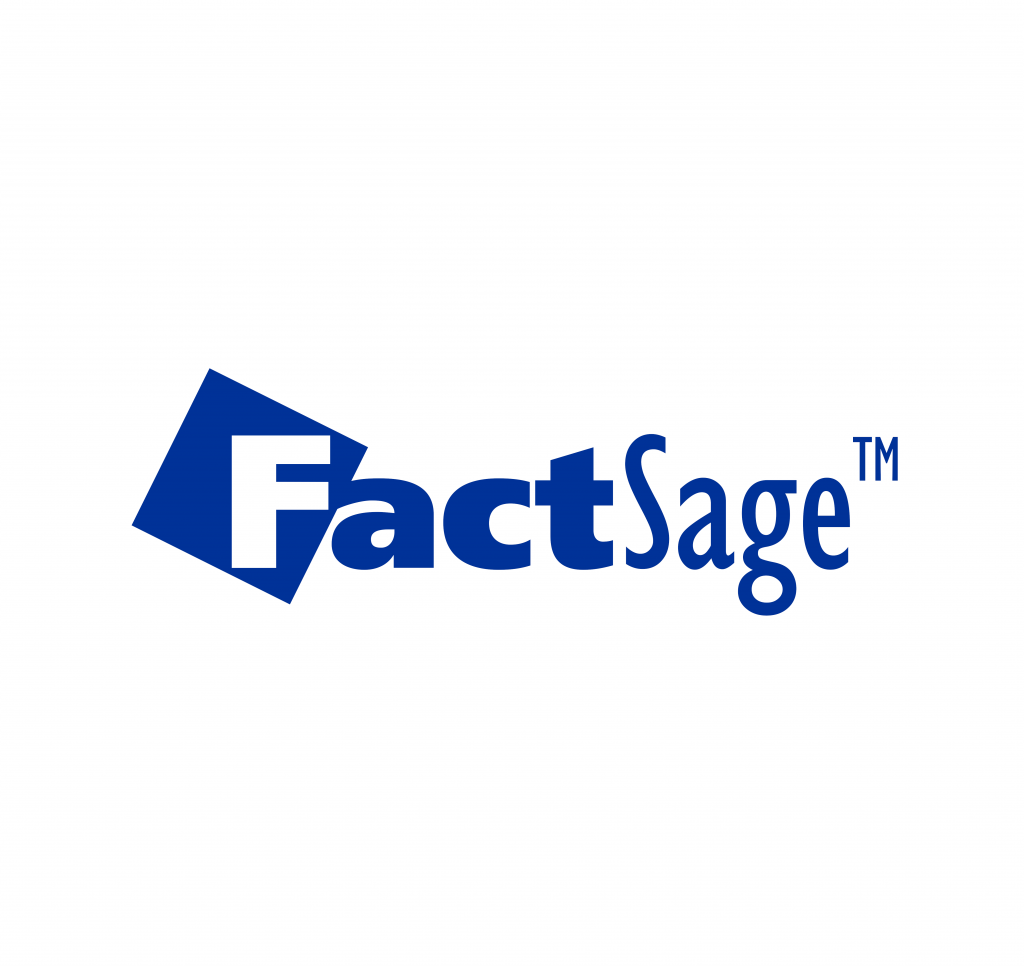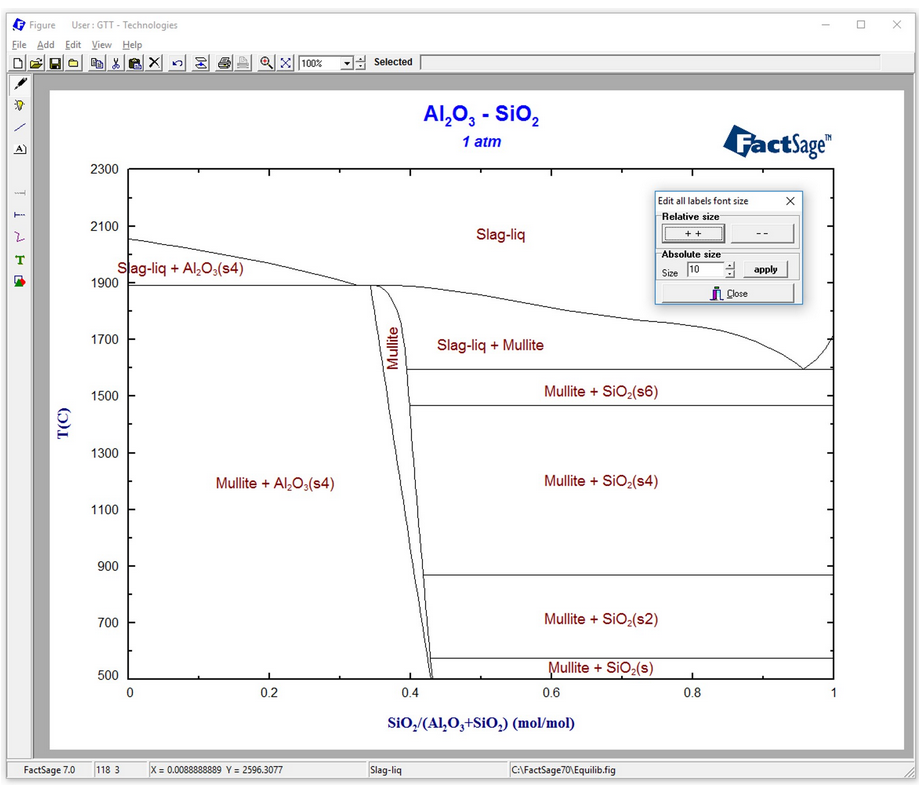# Phase diagram

## Minimization of copper losses to the converter slag through a boron oxide additionThe loss of copper in the converting process through its incorporation to the converter slag represents a major problem in the copper metallurgy. There are two important mechanisms for this phenomenon: the physicochemical and the mechanical one. The mechanical mechanism, i.e. the mechanical entrapment of matte or of metallic copper inside the slag, is by …

## 3D Mapping of Gibbs Energy Diagrams onto Phase DiagramsMats Hillert has shown [*] that what is called a Phase Diagram is derivable from a projection in a so-called Property Diagram. The (molar) Gibbs Energy as the property is plotted along the z-axis as a function of two other variables x and y. For binary systems, for example, T equals y and the mole …

## A hands-on example for a phase-diagram-module calculationFactSage contains many modules. The major ones for daily work are Equilib and Phase Diagram. Phase Diagram permits the calculation of phase diagram sections for systems with any number of components. Components can be elements, compounds or even arbitrary compositions. For a phase diagram calculation, first the components of interest have to be defined. Then, the possible …